Hello, readers welcome to the new post. In this post, we will discuss Power In Single Phase AC Circuits. The ac circuits are such circuits that use alternating current to operate and works.

In this post, we discuss the single-phase ac circuits and finds how power calculated in these circuits.

So let get started

### How to Finds Power In Single Phase AC Circuits

• In the case of energy transmission theory, there is energy transmission is discussed in form of electrical and magnetic fields.
• Normally the main factor discusses in energy transmission is the power that is a product of voltage and current.
• Power measured in watt
• Power consumed by the load in case of at any instant is multiple of instantaneous current and instantaneous voltage
• If load terminals are denoted with letters a and b then the value of voltage and current will be.

Vab=Vmaxcoswt and iab=imaxcos(wt-θ)

• the equation for instantaneous power will be

P=VabIab=Vmaxcoswtcos(wt-θ)

• If a load is capacitive then θ has a positive value when currents voltage and has a negative value when the current leads the voltage.
• The positive value for power will indicate that power is got by the system among the points a and b.
• the instantaneous power has positive value in case when Vab and Lab have positive value.
• This fact is explained here.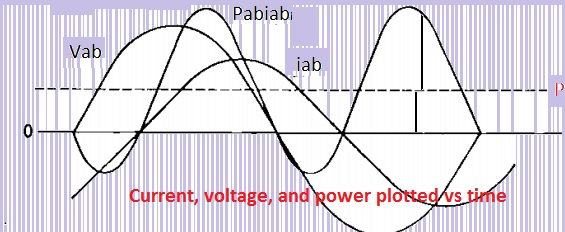• Positive power measured has to value Vab,Lab causes current to flow in direction of voltage loss and ratio of energy transfer for the load.
• While the negative value of power is measured when VabIab when the current direction si towards the high value of voltage. In other words, power is given to the load linked
• If the load is resistances that indicates that voltage and current are in phase so power will have a negative value
• While for loads that are inductive and capacitive the current and voltage have ninety-degree phase difference so instances power will be zero due to the same value of in both halves.
• Now above equation will become’

P=(VmImcosθ)/2(1+cos2wt)+(VmIm)/2sinθsin2wt

• In the above equation term (VmIm) can be replaced with multiple of rms voltage and current which has value ΙVΙiΙ
• there is another way to observe the equation for instantaneous power is to suppose that current is in phase tot the Vab
• In below figure you can see the parallel configured circuitry with a phasor diagram
• 1.3 RL Parallel Configured Circuit with phasor
• the component of Iab that is in phase to the Vab is iR and from the above phasor diagram, we can see that IR=Iabcosθ.
• Suppose the extreme value of iab is im so extreme value of IR is imcosθ

IR=cosθcoswt

• Here the element Iab lags voltage Vab through ninety degrees that is ix has value.
• Imsinθ as ix lagging the Vab through ninety degree we have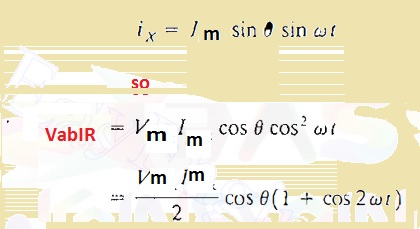• Now we have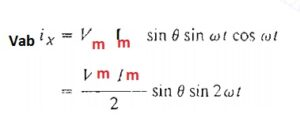• that is inatantoaoous power for inductacne
• the diagram indicate that Vab and Ix and their graph• Now we have

P=(VmIm)/2cosθ

### What is Complex Power

• If we know the equation for voltage and current then real and reactive power can be found through complex power shapes.
• If voltage and current are denoted as V=ΙVΙ∠α and I=ΙIΙ∠β
• the multiple of voltage and the current conjugate is given here.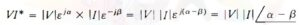• It is complex power and denoted with the letter S
• In the shape of a rectangle, we have an equation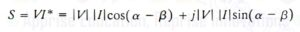S=P+JQ—–A

### What is the Power triangle

• Expression denoted with A indicates that phaser technique of getting the net value of P Q and phase angle in case of certain loads has parallel configuration since cosθ is P/S
• for inductvie load power trainagngle is consttruct.Tha is all bout Power In Single Phase AC Circuits if you have any further query ask in the comments. Thanks for reading have a nice day